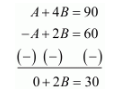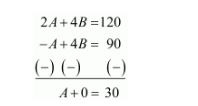Deepak Scored 45->99%ile with Bounce Back Crack Course. You can do it too!

# Find acute angles A and B, if sin (A+2B)=3√2 and cos (A+4B)=0, A>B.

Question:

Find acute angles $\mathrm{A}$ and $\mathrm{B}$, if $\sin (A+2 B)=\frac{\sqrt{3}}{2}$ and $\cos (A+4 B)=0, A>B$

Solution:

Given:

$\sin (A+2 B)=\frac{\sqrt{3}}{2}$....(1)

$\cos (A+4 B)=0$....(2)

We know that,

$\sin 60^{\circ}=\frac{\sqrt{3}}{2}$....(3)

$\cos 90^{\circ}=0$....(4)

Now by comparing equation (1) and (3)

We get,

$A+2 B=60 \ldots \ldots(5)$

Now by comparing equation (2) and (4)

We get,

$A+4 B=90 \ldots \ldots(6)$

Now to get the values of A and B, let us solve equation (5) and (6) simultaneously

Therefore by subtracting equation (5) from (6)

We get,Therefore,

$2 B=30$

$\Rightarrow B=\frac{30}{2}$

$\Rightarrow B=15^{\circ}$

Hence $B=15^{\circ}$

Now by multiplying equation (5) by 2

We get,

$2 A+2 \times 2 B=2 \times 60$

$2 A+4 B=120 \ldots \ldots(7)$

Now by subtracting equation (6) from (7)

We get,Therefore,

$A=30$

Hence $A=30^{\circ}$

Therefore the values of $A$ and $B$ are as follows $A=30^{\circ}$ and $B=15^{\circ}$